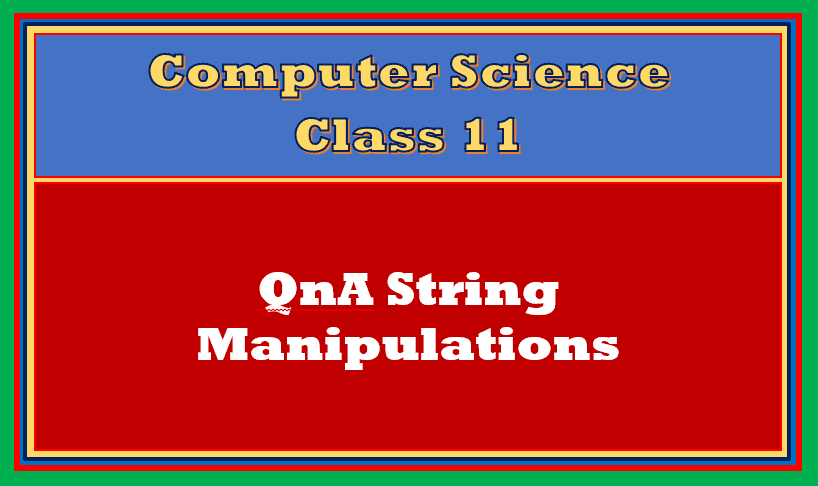If you are looking for important QnA String Manipulation in Python Class 11. Enjoy this article!

If you missed earlier QnA Articles, please refer the below given link:

Topics Covered

## Objective type questions

#### Fill in the blanks

1. The data or text enclosed with single quote, double quote or triple quote is known as _____________.
2. The string which is having 0 characters is known as ___________.
3. Each character in a string has a unique position or ID in the text, that is known as ___________.
4. The index of string is start from _______ to ___________ in forward direction.
5. The process of accessing a string character by character is known as __________.
6. The ___________ operator is used to repeat the word or specified text n times.
7. To join more than two words you can use __________ operator.
8. The ______________ operator returns True if a character or specified atring is available in the given string.
9. The _________ and ____________ operators are membership operators.
10. To reverse a string using string slice, __________ is an easy way to do so.

1. String
2. Empty String
3. Index
4. 0, length-1
5. Traversing
1. * (Replication)
2. + (Concatenation)
3. in
4. in, not in
5. s[::-1]

The next section of QnA String Manipulation in Python Class 11 provides MCQs from the topic.

#### Multiple Choice Questions

 Which of the following is the correct way of indexes of string, begin from the reverse in backward direction?

i) 0 to Length – 1

ii) -1 to -length

iii) -length to – 1

iv) Length -1 to 0

a) i), ii) and iii)

b) ii) and iv)

c) Only Option ii)

d) ii), iii) and iv)

 Iterating through the various elements of a string, one character at a time is known as ____________

a) String Indexing

b) String Traversing

c) String Replicating

d) None

 When you write S[-5] for a five letter word, python will return

a) S

b) S

c) S

d) S[-5]

 What will be the result of this code: ‘2’ + 3

a) 5

b) 23

c) Error

d) 1

 What will be the result of this code: ‘*’ * 3

a) Error

b) *3

c) ***

d) 3*

 The expression “CSIP” in “TutorialAICSIP” returns

a) Found

b) True

c) 0

d) 1

 The expression “Tutor” <=”TutorialAICSIP” returns

a) 1

b) True

c) 0

d) False

 Which of the following function returns the ASCII code for specified character?

a) ASCII()

b) asc()

c) ord()

d) code()

 Which of the following function returns the character from given integer code?

a) char()

b) ord()

c) cha()

d) chr()

 What will be the result of s[len(s),-3]

a) Return 3 letters from reverse

b) Error

c) First 3 letters of string

d) None of these

 To print first 4 letters from the string, which of the option(s) is/are correct?

i) s[:3]

ii) s[0:4]

iii) s[:4]

iv) s[0:3]

a) Option i) and iv)

b) Option ii) and iii)

c) Option ii) Only

d) Option iii) only

 Which of the following is not a correct string operation in python?

a) ‘Tut’ + ‘or’

b) ‘Tutor’ * 2

c) ‘Tutor’ + 2

d) 2 * ‘Tutor’

1. b) ii) and iv)
2. b) String Traversing
3. S
4. c) Error
5. c) ***
6. b) True
7. b) True
8. c) ord()
9. d) chr()
10. b) Error
11. b) Option ii) and iii)
12. c) ‘Tutor’ + 2

In the next section of QnA String Manipulation in Python Class 11, we will give you some questions. To find the answers read our recommended article.

1. What do you mean by a string? How it differs from other data types?
2. Justify the statement – “Python strings are immutable”.
3. What do you mean by index? What is the key role of indexes in a python string?
4. What do you mean by traversing? Elaborate your answer with an example.
5. Write code to print alternate characters of the given string in the output.
6. What are the differences between + and * operator in the string?
7. How to work with ASCII values in python? Explain all the possible ways or functions deals with ASCII values in python. Support your answer with at least one example of each.
8. Explain the following string slices in your words with appropriate examples:
1. [:]
2. [::]
3. [5:]
4. [:5]
5. [::-1]
6. [5:0]
7. [5::-1]
8. [5:-5]
9. 

In the next section of QnA String Manipulation in Python Class 11 we will discuss output based questions.

### Output Based Questions

Observe the following code and predict the output:

 Code 1:

``````print("""
T
U
T
O
R
I
A
L
A
I
C
S
I
P
""")``````

 Code 2:

``````a = str("786")
b = "Python" * 4
print(a,b)
a = a + b
print(len(a))``````

 Code 3:

``````s="python string fun"
for i in range(0,len(s)):
if s[i]>='c' and s[i]<='l':
print(s[i].lower(),end="")
else:
print(s[i].upper(),end="")
``````

 Code 4:

``````s="python string fun"
while True:
if s=='p':
s = s[3:]
elif s[-1]=='n':
s=s[:2]
else:
break
print(s)
``````

 Code 5:

``````s="python"
s1=""
s2=""
s3=""
if s[-1]=='n':
s1 = s[0:3]
print(s1)
if 'h' in s:
s2=s + 'i'
print(s2)
if 'z' not in s:
s3 = '7' + s[1:] + 'w'
print(s3)
else:
s = s* 3
print(s)
``````